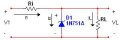# Zener Dioide Problem

#### Digit0001

Joined Mar 28, 2010
100
Hi

How would you do the following problem:

A Zener diode is connected in a voltage regulator circuit.The Zener voltage is Vz = 10V and the Zener resistance is assumed to be rz=0. (a) Determine the value of Ri such that the Zener dioide remains in breakdown if the load current vari4es from Iload =50 to 500mA and if the input voltage varies from V1 = 15 to 20V. Assume Iz(min) = 0.1I(max).

P.S

#### t_n_k

Joined Mar 6, 2009
5,455
The constraints are minimal.

The minimum zener current would presumably occur when the input voltage is at its lowest and the load is at its maximum. You are advised to set Iz(min) to 0.1Iload(max). That should be sufficient information to solve the problem.

#### Digit0001

Joined Mar 28, 2010
100
i have tried doing this:

I1= Iz+Il

Iz = Vz/rz = 0

so I1 = IL

now (Vin(min) - Vz)/ Ri = I1 = IL(min)

(20 - 10)/ Ri = 50m

10/50m = Ri

so Ri = 200ohms

checked with answer this is wrong, what is wrong with this??

#### MrChips

Joined Oct 2, 2009
29,848
At max Vi Ri = 10/.5 = 20 ohm
If you need to include Iz, Rz = 200 ohm
Then Ri = 20//200 = 200/11 = 18 ohm

#### t_n_k

Joined Mar 6, 2009
5,455
It might be worth double checking that Ri=18Ω actually works.

#### jimkeith

Joined Oct 26, 2011
540
Ri = ERimin /IRi
ERi min = 15-10 = 5V
IRi under above conditions = 0.5 + 0.1*0.5 = 0.55A
Ri = 9.09Ω

A better question is: Specify a zener that will do this without smoke

#### t_n_k

Joined Mar 6, 2009
5,455
Ri = ERimin /IRi
ERi min = 15-10 = 5V
IRi under above conditions = 0.5 + 0.1*0.5 = 0.55A
Ri = 9.09Ω

A better question is: Specify a zener that will do this without smoke
Agree with your value for Ri - hence my query.

Excellent point about the practical implications.